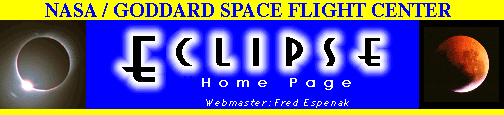# Total Solar Eclipse of 2001 June 21

## Table 1 - Elements of the Eclipse```
Geocentric Conjunction      11:58:54.06 TDT     J.D. = 2452081.999237
of Sun & Moon in R.A.:   (=11:57:49.06 UT)

Instant of            12:04:46.26 TDT     J.D. = 2452082.003313
Greatest Eclipse:      (=12:03:41.26 UT)

Geocentric Coordinates of Sun & Moon at Greatest Eclipse (DE200/LE200):

Sun:       R.A. = 06h00m46.131s       Moon:      R.A. = 06h01m00.453s
Dec. =+23°26´18.20"                   Dec. =+22°52´27.17"
Semi-Diameter =    15´44.26"          Semi-Diameter =    16´17.69"
Eq.Hor.Par. =       08.65"            Eq.Hor.Par. =  0°59´47.93"
delta R.A. =       10.406s/h          delta R.A. =      156.807s/h
delta Dec. =       -0.18"/h           delta Dec. =      196.15"/h

Lunar Radius   k1 = 0.2725076 (Penumbra)        Shift in      delta b =  0.00"
Constants:    k2 = 0.2722810 (Umbra)       Lunar Position:   delta l =  0.00"

Geocentric Libration:   l =  -3.8°       Brown Lun. No. =  971
(Optical + Physical)    b =   0.7°         Saros Series =  127 (57/82)
c =   2.0°            Ephemeris = (DE200/LE200)

Eclipse Magnitude = 1.04953        Gamma =-0.57014        delta T =    65.0 s

xxxxxxxxx
Polynomial Besselian Elements for:   2001 Jun 21   12:00:00.0 TDT  (=t0)

n        x          y         d          l1         l2          Á

0   0.0103551 -0.5718306 23.4397678  0.5369922 -0.0091193 359.564514
1   0.5653870  0.0551258 -0.0001831 -0.0000944 -0.0000939  14.999194
2   0.0000292 -0.0001339 -0.0000056 -0.0000121 -0.0000121   0.000000
3  -0.0000089 -0.0000010  0.0000000  0.0000000  0.0000000   0.000000

Tan f1 = 0.0046005    Tan f2 = 0.0045776

At time "t1" (decimal hours), each Besselian element is evaluated by:

a = a0 + a1*t + a2*t2 + a3*t3    (or a = À [an*tn]; n = 0 to 3)

where:  a = x, y, d, l1, l2, or Á
t = t1 - t0  (decimal hours) and t0 = 12.000 TDT

The Besselian elements were derived from a least-squares fit to elements
calculated at five separate times over a six hour period centered at t0.
Thus the Besselian elements are valid over the period 9.00 < t0 < 15.00 TDT.

Saros Series 127:  Member 57 of 82 eclipses in series.

```

Table adapted from NASA TP 1999-209484 "Total Solar Eclipse of 2001 June 21".WebMaster: Fred Espenak
Planetary Systems Branch - Code 693
e-mail: espenak@lepvax.gsfc.nasa.gov

NASA/Goddard Space Flight Center, Greenbelt, Maryland 20771 USALast revised: 1999 Dec 09 - F. Espenak